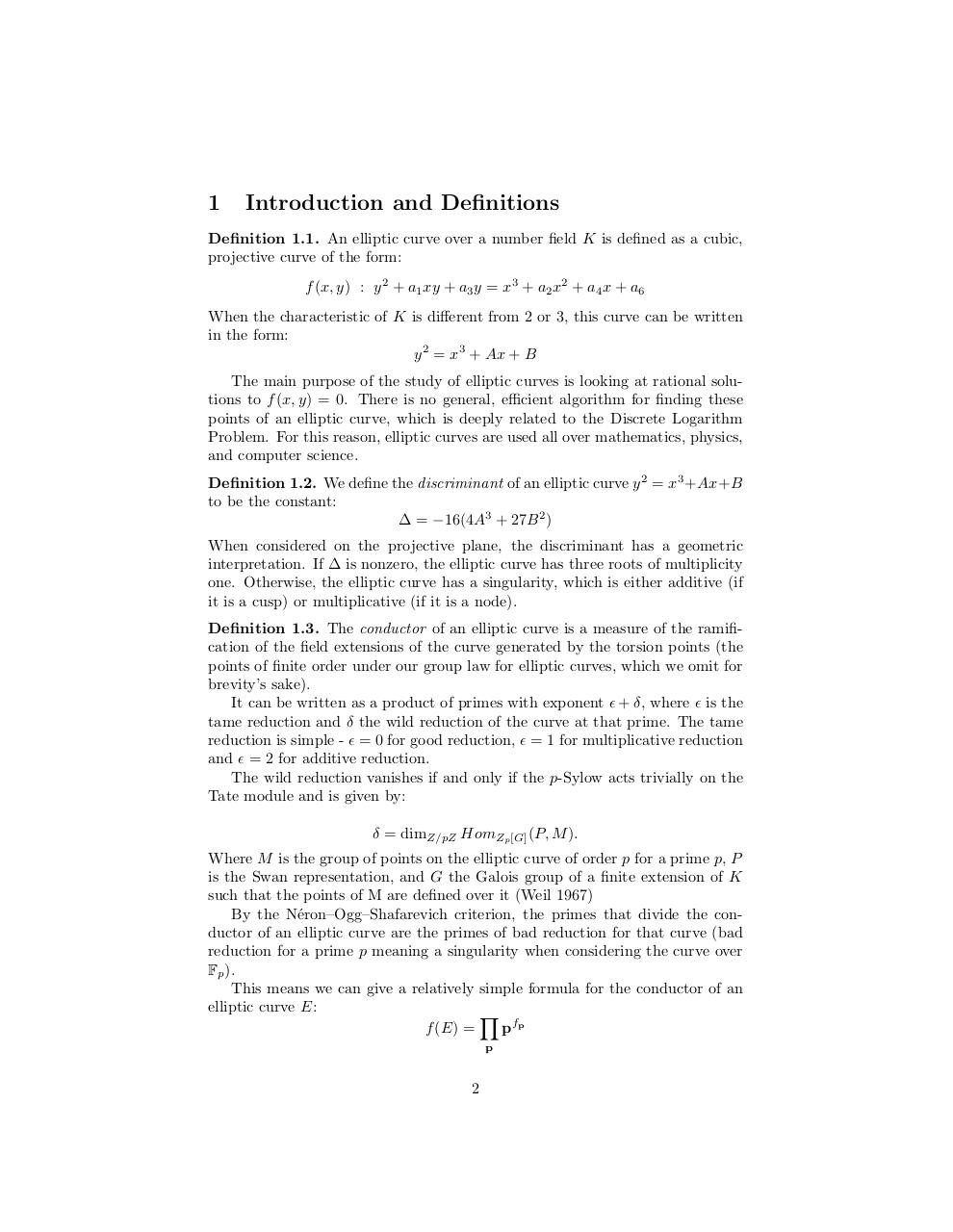# Sci Research (2).pdfPage 1 2 3 4 5 6 7 8 9

#### Text preview

1

Introduction and Definitions

Definition 1.1. An elliptic curve over a number field K is defined as a cubic,
projective curve of the form:
f (x, y) : y 2 + a1 xy + a3 y = x3 + a2 x2 + a4 x + a6
When the characteristic of K is different from 2 or 3, this curve can be written
in the form:
y 2 = x3 + Ax + B
The main purpose of the study of elliptic curves is looking at rational solutions to f (x, y) = 0. There is no general, efficient algorithm for finding these
points of an elliptic curve, which is deeply related to the Discrete Logarithm
Problem. For this reason, elliptic curves are used all over mathematics, physics,
and computer science.
Definition 1.2. We define the discriminant of an elliptic curve y 2 = x3 +Ax+B
to be the constant:
∆ = −16(4A3 + 27B 2 )
When considered on the projective plane, the discriminant has a geometric
interpretation. If ∆ is nonzero, the elliptic curve has three roots of multiplicity
one. Otherwise, the elliptic curve has a singularity, which is either additive (if
it is a cusp) or multiplicative (if it is a node).
Definition 1.3. The conductor of an elliptic curve is a measure of the ramification of the field extensions of the curve generated by the torsion points (the
points of finite order under our group law for elliptic curves, which we omit for
brevity’s sake).
It can be written as a product of primes with exponent  + δ, where  is the
tame reduction and δ the wild reduction of the curve at that prime. The tame
reduction is simple -  = 0 for good reduction,  = 1 for multiplicative reduction
and  = 2 for additive reduction.
The wild reduction vanishes if and only if the p-Sylow acts trivially on the
Tate module and is given by:
δ = dimZ/pZ HomZp [G] (P, M ).
Where M is the group of points on the elliptic curve of order p for a prime p, P
is the Swan representation, and G the Galois group of a finite extension of K
such that the points of M are defined over it (Weil 1967)
By the N´eron–Ogg–Shafarevich criterion, the primes that divide the conductor of an elliptic curve are the primes of bad reduction for that curve (bad
reduction for a prime p meaning a singularity when considering the curve over
Fp ).
This means we can give a relatively simple formula for the conductor of an
elliptic curve E:
Y
f (E) =
pfp
p

2